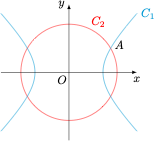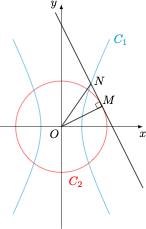# 每日一题隐藏的切线1、若 $x_{A}=\sqrt{6}$，求 $b$．

2、若 $b=\sqrt{5}$，圆与 $x$ 轴交点分别为 $F_{1}, F_{2}$，点 $P$ 在双曲线第一象限部分，且 $\left|P F_{1}\right|=8$，求 $\angle F_{1} P F_{2}$．

3、过点 $S\left(0, \dfrac{b^{2}}{2}+2\right)$ 斜率为 $-\dfrac{b}{2}$ 的直线 $l$ 与曲线 $\Gamma$ 交于 $M,N$ 两点，用 $b$ 表示 $\overrightarrow{O M} \cdot \overrightarrow{O N}$ 并求其取值范围．

1、若 $x_A=\sqrt 6$，则$\begin{cases} \dfrac{x_A^2}4-\dfrac{y_A^2}{b^2}=1,\\ x_A^2+y_A^2=4+b^2,\end{cases}\iff \begin{cases} b^2=4,\\ y_A^2=2,\end{cases}$于是 $b=2$．

2、当 $b=\sqrt 5$ 时，有 $C_1:\dfrac{x^2}4-\dfrac{y^2}5=1$，$C_2:x^2+y^2=9$，此时 $F_1(-3,0)$，$F_2(3,0)$，为双曲线的左、右焦点．由 $|PF_1|=8$ 和双曲线的定义可得 $|PF_2|=4$，而 $|F_1F_2|=4$，在 $\triangle F_1PF_2$ 中应用余弦定理，可得$\cos\angle F_1PF_2=\dfrac{|PF_1|^2+|PF_2|^2-|F_1F_2|^2}{2\cdot |PF_1|\cdot |PF_2|}=\dfrac{11}{16},$于是 $\angle F_1PF_2=\arccos\dfrac{11}{16}$．

3、根据题意，直线 $l$ 的方程为 $y=-\dfrac b2x+\dfrac {b^2}2+2$，即 $bx+2y-b^2-4=0$，因此直线 $l$ 是圆 $C_2$ 的切线，且切点坐标为 $(b,2)$．